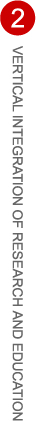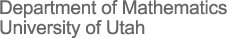# Friday April 26 Session - 2pm-4pm - LCB 323

02:00pm-02:20pm, Alessandro Gondolo, Characterization and Synthesis of Rayleigh-damped elastodynamic networks
Mentor: Fernando Guevara Vasquez
Abstract: We consider damped elastodynamic networks where the damping matrix is assumed to be a non-negative linear combination of the stiffness and mass matrices (Rayleigh damping). We give here a characterization of the frequency response of such networks. We also answer the synthesis question for such networks, i.e., how to construct a Rayleigh damped elastodynamic network with a given frequency response. The characterization and synthesis questions are sufficient and necessary conditions for a frequency dependent matrix to be the frequency response of a Rayleigh damped network. We only consider the non-planar case.

02:20pm-02:40pm, Ben DeViney, Suction Feeding on Multiple Prey
Mentor: Tyler Skorczewski
Abstract: Suction feeding is one of the most common forms of predation among aquatic feeding vertebrates. There are several instances in nature and technology that show numerous bodies in close proximity working together to reduce the effects of drag. Here we simulate a suction feeding attack on multiple prey items in an attempt to understand how the fluid dynamics changes between single and multiple prey cases. To model a suction feeding attack, we numerically find solutions to the incompressible Navier-Stokes equations on Chimera overset grids. In comparing the prey capture times across various cases, our results show that additional prey have no effect. From these results, we can conclude that suction feeding mechanisms are precise and highly localized to the point where multiple prey items have no effect on the suction attack.

02:40pm-03:00pm, Alex Burringo, Carli Edwards, Trevor Myrick, Mathematical Modeling of Molecular Motors
Mentors: Parker Childs and Ross Magi
Abstract: Kinesin and dynein are molecular motor proteins that transport cargo in opposite directions along microtubules in cells. They are of interest in biophysics because of their roles in DNA replication and intracellular transport. Despite considerable experimental research concerning single motor dynamics, a clear theoretical explanation for motor procession along cellular microtubule filaments is not present, especially when many motors are present. Current research shows that it is common for multiple dynein and kinesin motors to be attached to a single cargo. However, there is much speculation as to how the cargo moves given this expected tug-of-war possibility. To help reconcile current theories for procession, we created a stochastic tug-of-war model of kinesin and dynein dynamics to simulate data for comparison with current experimental data. Much of the semester was spent coding the molecular movement of dynein and kinesin, with evaluation of stepping probabilities, diffusion force effects, cargo-motor attachment forces, effect of multiple motors, motor-microtubule attachment forces, and the addition of temperature variations. Because of the stochastic nature of the code, it was run numerous times in parallel on a math department compute cluster. Evaluation of model effectiveness will be done by statistical comparison of the simulated and experimental results, specifically by Dr. Vershinin in the Physics Department, to aid in the continuing research of molecular motor dynamics.

Mentor: Gordan Savin
Abstract: Binary quadratic forms are quadratic forms in two variables having the form $f(x, y) = ax^2 + bxy + cy^2$. In this talk we'll discuss the reduction, automorphisms, and finiteness reduced forms of a given discriminant. The main objective will be to present an interesting proof about the relationship between automorphisms of forms and solutions to the Pell equation.

03:20pm-03:40pm, Steven Sullivan, A Trace Formula for $G_2$
Mentor: Gordan Savin
Abstract: An $n$-dimensional representation of a group $G$ on a vector space $V$ is a homomorphism from $G$ to $GL(V)$. For our purposes, we consider an irreducible representation to be a representation which cannot be decomposed into the direct sum of smaller-dimensional representations. Let $H$ be a subgroup of $G$. The way in which irreducible representations of $G$ decompose into irreducible representations of $H$ is called branching. In order to calculate such branching, one must first obtain a trace formula for each conjugacy class of $H$ in irreducible representations of $G$. In this talk, we summarize the obstacles which must be overcome when attempting to calculate such trace formulas for the conjugacy classes of $G_2(2)$ in irreducible representations of $G_2$. Then we show the methods we used to overcome these challenges and find the trace formulas for the sixteen conjugacy classes.

03:40pm-04:00pm, Wyatt Mackey, On the inverse Galois problem for symmetric groups and quaternions
Mentor: Dan Ciubotaru
Abstract: The Galois group was introduced in relation to the question of solvability by radicals of polynomial equations. The inverse Galois problem asks if, given a finite group $G$, there exists a field extension of rational numbers such that the Galois group equals $G$. This projects investigate explicit field extensions when $G$ is the quaternionic group $Q_8$ or a symmetric group.

# Monday April 29 Session - 2pm-4pm - JWB 333

02:00pm-02:20pm, Sean Quinonez, Street fighters: a model of conflict in Tetramorium caespitum
Abstract: Mathematical models provide a powerful tool for describing and analyzing interactions between populations of organisms. Of interest are interactions among eusocial populations, where simple rules at the individual level translate into complex patterns of interaction within and between colonies. We present a model that describes conflict between colonies of the ant Tetramorium caespitum, an ideal study species due to their abundance and status as an urbanized species. Conflicts between T. caespitum colonies arise during seasonal competition for territory. Our mathematical model tracks flow of ants in each of two colonies as they recruit and travel to a conflict, where ants search and grapple with enemies. The model will examine two mechanisms of motivation, and study how these mechanisms control the location and size of the battle. These recruitment mechanisms introduce delays that can produce oscillations in the location and make-up of the battle, model predictions that correspond to empirical measurements of ant battles.

02:20pm-02:40pm, Skip Fowler, Mathematical modeling of epidemics using a probabilistic graph
Abstract: In order to predict the number of survivors, we study a stochastic model of an epidemic that includes with transitions infection and recovery. This approach can be represented as a graph with probabilities on the edges between states. The distribution of the number of survivors can be found from the set of probabilities associated with states where the number of infected people equals $0$. We find that this probability distribution breaks into two distinct components. We compute the probability of rapid epidemic extinction with many survivors, $Q$, using methods from stochastic process theory. If the epidemic takes hold, we use an SIR model without vital dynamics, a deterministic set of differential equations describing the spread of an epidemic, which approaches zero only when the epidemic begins and ends. We compare the mean of the probabilities for states after $Q$ to determine if the mean matches the end point of the epidemic in the deterministic SIR model, and check whether their distribution is approximately normal. We developed computer simulations to calculate and plot the probabilities and compare with the mathematical analysis of $Q$ and the SIR model. Our discoveries during the course of this project include finding the Catalan numbers and Catalan's triangle within the graph structure and finding a knights move based on the number of steps to any state within the graph structure. The probabilities after $Q$ are not normal due to increasing kurtosis as population size and infection rate are increased, although the mean is well-approximated by the differential equation. We are searching for a higher dimensional system that captures this deviation from normality.

02:40pm-03:00pm, Kyle Zortman, Modeling the Progression to Invasive Cervical Cancer
Abstract: This talk will discuss the biological development of cervical cancer in the body and the difficulties of stochastically modeling this process. Because progression of cervical cancer is time and stage dependent, simple stochastic modeling approaches fail. A solution is then proposed using a modification of the Gillespie Algorithm. Simulation will be compared to real data and more deterministic solutions. Finally, the system will be analyzed for sensitivity and applications will be discussed.

03:00pm-03:20pm, Boya Song, Horizontal fluid transport through Arctic melt ponds
Mentor: Ken Golden
Abstract: The albedo or reflectance of the sea ice pack is an important parameter in climate modeling. Being able to more accurately predict sea ice albedo can significantly increase the reliability of climate model projections. Sea ice albedo is closely related to the evolution of melt ponds on the surface of Arctic sea ice. In the process of melt pond evolution, a critical component is the drainage of surface melt water through holes and cracks that exist naturally in ice floes. The speed and range of the drainage is related to how easy it is for fluid to flow horizontally over the ice. This horizontal fluid conductivity can be modeled using random resistor networks. The horizontal conductivity of the melt pond configurations plays a key role in modeling melt pond evolution, and therefore sea ice albedo. In this lecture we will discuss efforts at mapping melt pond configurations onto random graphs, and then developing efficient algorithms to calculate the effective conductivity of these random graphs.

03:20pm-03:40pm, Brady Bowen, Random Fourier Surfaces: Applications to Arctic Melt Pond Modeling
Mentor: Ken Golden
Abstract: Arctic melt ponds are important in climate models because they modify the level of reflectance, or albedo, of the sea ice pack. We decided to model this system using a two dimensional random Fourier series expansion. Level sets of these random surfaces give shapes that closely resemble the melt ponds found in the Arctic. Random Fourier surfaces also have multiple controlled variables that can be changed to determine which length scale dominates the generated surface. We modified these constants in order to obtain a better fitting match of the observed melt ponds. We then calculated area-perimeter data in order to find at what point the generated shapes went through a fractal dimension transition, which is exhibited by real data obtained from the Arctic melt ponds. It was also found that changing the variables which characterize the Fourier surfaces altered where this fractal dimension shift occurred and how fast the fractal dimension of the shapes changed.